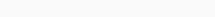# Math Puzzles Puzzle 63 Answer AndroidMath puzzle game level 63 solution with detailed hints and explanation, Scroll below to find out.

Math puzzles increase your logical thinking. The objective of the game is to find patterns hidden in the puzzle in order to arrive at the solution. Challenge yourself with different levels of math puzzles and stretch the limits of your intelligence.

### Math Puzzles Puzzle 63:

3 3 1=4

5 1 3=6

7 2 4=7

8 3 7=9

then 9 1 9=?Solution/Hint: 3+13=16=4

31+5=36=6

42+7=49=7

73+8=81=9

91+9=100=10

If there is any Doubt or you are unable to understand the solution, then please let me know in comments, I will surely help you.

### 4 thoughts on “Math Puzzles Game Level 63 Answer with Solution”

•March 15, 2019 at 08:32

Sqrt(cb+a)=

•January 1, 2023 at 10:34

Ca+b

•February 16, 2019 at 16:09

What dont 16 as follow
3-1 =2/3-1=2/2+2=4
5-1=4/3-1=2/4+2=6
7-2=5/4-2=2/5+2=7
8-3=5/7-3=4/5+4=9
Then 9-1=8/9-1=8/8+8=16

•